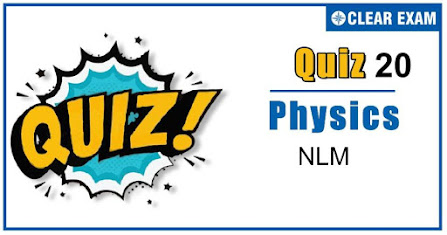## [LATEST]\$type=sticky\$show=home\$rm=0\$va=0\$count=4\$va=0

As per analysis for previous years, it has been observed that students preparing for NEET find Physics out of all the sections to be complex to handle and the majority of them are not able to comprehend the reason behind it. This problem arises especially because these aspirants appearing for the examination are more inclined to have a keen interest in Biology due to their medical background. Furthermore, sections such as Physics are dominantly based on theories, laws, numerical in comparison to a section of Biology which is more of fact-based, life sciences, and includes substantial explanations. By using the table given below, you easily and directly access to the topics and respective links of MCQs. Moreover, to make learning smooth and efficient, all the questions come with their supportive solutions to make utilization of time even more productive. Students will be covered for all their studies as the topics are available from basics to even the most advanced. .

Q1. An automobile travelling with a speed of 60 km/h, can brake to stop within a distance of 20 m. If the car is going twice at fast,i.e. 120 km/h, the stopping distance will be
•  20 m
•  40 m
•  60 m
•  80 m
Solution
The stopping distance, S∝u2 (∵v2=u2-2as) ⇒S2/S1 =u2/u12=120/602=4 ⇒S2=4×S1=4×20=80 m

Q2. A block is lying static on the floor. The maximum value of static frictional force on the block is 10N. If a horizontal force of 8 N is applied to the block, what will be the frictional force on the block
•  2 N
•  18 N
•  8 N
•  10 N
Solution

The limiting force of friction is fs=10 N As F=8Nfs,therefore, block does not move. As static friction is a self adjusting force, therefore the frictional force on the block is 8 N

Q3. Two masses A and B of 10 kg and 5 kg respectively are connected with a string passing over a frictionless pulley fixed at the corner of a table as shown. The coefficient of static friction of A with table is 0.2. The minimum mass of C that may be placed on A to prevent it from moving is
•  15 kg
•  10 kg
•  5 kg
•  12 kg
Solution
For limiting condition μ=mB/mA+mC⇒0.2=5/10+mC ⇒2+0.2mC=5⇒mC=15 kg

Q4. A body is under the action of two mutually perpendicular forces of 3 N and 4 N. The resultant force acting on the body is
•  7 N
•  1 N
•  5 N
•  Zero
Solution
Resultant force, F=√F12+F22+2F1 F2cos⁡θ =√32+42+2×3×4 cos⁡90° =5 N

Q5. In the figure a smooth pulley of negligible weight is suspended by a spring balance. Weights of 1 kg and 5 kg are attached to the opposite ends of a string passing over the pulley and move with acceleration because of gravity. During their motion, the spring balance reads a weight of
•  6 kg
•  Less than 6 kg
•  More than 6 kg
•  May be more or less than 6 kg
Solution
Spring balance reading in terms of kgf =4m1 m2/m1+m2=(4×5×1)/6=10/3 This is less than 6 kgf

Q6. A mass of 100 g strikes the wall with speed 5 ms-1 at an angle as shown in figure and it rebounds with the same speed. If the contact time is 2×10-3) s, what is the force applied?
•  250√3 N to right
•  250 N to right
• 250√3 N to left
•  250 N to left
Solution

Change in the velocity =v sin⁡θ--v sin⁡θ=2 sin⁡θ Change in the momentum ∆p=2 mv sin⁡θ ∴ Force applied F=∆p/∆t =2×100×10-3×5 sin⁡θ 60°/2×10-3 =100×5×√3/2 =250√3 N (To the right)

Q7. A block of mass M is attached to the lower end of a vertical spring. The spring is hung from a ceiling and has force constant value k. The mass is released from rest with the spring initially unstretched. The maximum extension produced in the length of the spring will be
•  1 Mg/k
•  2Mg/k
•  4 Mg/k
•  Mg/2k
Q8. A particle moves in the x-y plane under the influence of a force such that its linear momentum is p ⃗(t)=A[i ̂cos⁡(kt)-j ̂sin⁡(kt)] Where Aand k are constants. The angle between the force and momentum is
•
•  30°
•  45°
•  90°
Solution
p ⃗(t)=Ai ̂cos⁡kt-j ̂sin⁡kt F ⃗=d/dt (p ⃗(t) )=Ak-i ̂sin⁡kt-j ̂cos⁡kt)
F ⃗.p ⃗=A2 k-cos⁡kt sin⁡kt+sin⁡kt cos⁡kt=0 ∴ The momentum and force are perpendicular to each other at 90°

Q9.  An open carriage in a goods train is moving with a uniform velocity of 10ms-1. If the rain adds water with zero velocity at the rate of 5 kgs-1, then the additional force applied by the engine to maintain the same velocity of the train is
•  0.5 N
•  2.0 N
•  50 N
•  25 N
Solution
F=v dm/dt=10×5 N = 50 N

Q10. A marble block of mass 2 kg lying on ice when given a velocity of 6 ms-1 is stopped by friction in 10 s. Then the coefficient of friction is
•  0.02
•  0.03
•  0.06
• 0.01
Solution
Let coefficient of friction isμ, and then retardation will be μg. From equation of motion, v=u+at ⟹ 0=6-μ g×10 ⟹μ= 6/100=0.06## Want to know more

Please fill in the details below:

## Latest NEET Articles\$type=three\$c=3\$author=hide\$comment=hide\$rm=hide\$date=hide\$snippet=hide

Name

ltr
item
BEST NEET COACHING CENTER | BEST IIT JEE COACHING INSTITUTE | BEST NEET & IIT JEE COACHING: NLM Quiz 20
NLM Quiz 20
https://1.bp.blogspot.com/-Un4Q-3j276s/X5aGrSF7AQI/AAAAAAAABng/7SqV47zNn2I1oHZ1fbPr4JK9vCqXbSD8wCLcBGAsYHQ/w447-h235/Quiz%2BImage%2BTemplate%2B%252819%2529.jpg
https://1.bp.blogspot.com/-Un4Q-3j276s/X5aGrSF7AQI/AAAAAAAABng/7SqV47zNn2I1oHZ1fbPr4JK9vCqXbSD8wCLcBGAsYHQ/s72-w447-c-h235/Quiz%2BImage%2BTemplate%2B%252819%2529.jpg
BEST NEET COACHING CENTER | BEST IIT JEE COACHING INSTITUTE | BEST NEET & IIT JEE COACHING
https://www.cleariitmedical.com/2020/10/NLMQuiz20.html
https://www.cleariitmedical.com/
https://www.cleariitmedical.com/
https://www.cleariitmedical.com/2020/10/NLMQuiz20.html
true
7783647550433378923
UTF-8

STAY CONNECTED